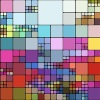#### You may also like### Sine Problem

In this 'mesh' of sine graphs, one of the graphs is the graph of the sine function. Find the equations of the other graphs to reproduce the pattern.### Squaring the Rectangle

Can you find a way to turn a rectangle into a square?### The Use of Mathematics in Computer Games

An account of how mathematics is used in computer games including geometry, vectors, transformations, 3D graphics, graph theory and simulations.

# Footprints

##### Age 16 to 18Challenge Level

To find the formula for this combinaton of three reflections requires very little knowledge of complex numbers. All you have to do is to combine the complex maps as you combine functions.. The example shows the power of complex numbers as a tool for working with transformations in the plane.

In the problem Complex Rotations you see another examle of using complex numbers for work with transformations.

The problems Rots and Refs and Reflect Again are examples of the use of matrices for work with transformations.

This problem is about combinations of reflections. The problem Reflect Again uses matrices to show that the combination of two reflections in intersecting mirror lines gives a rotation.